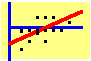8.1 Overview

The Markowitz model is an elegant formulation of the portfolio problem that forms the basis of the Capital Asset Pricing Model (CAPM). From a practical point of view, however, it has two shortcomings. The first is the problem of computational complexity. To see this, consider that over 2000 stocks are traded on the NYSE alone. The covariance matrix of returns is a 2000x2000 matrix, which contains 4 million numbers (though about half of these are the same). Imagine first estimating all the parameters in this matrix, and then using the matrix to calculate the efficient frontier. Now, add the thousands of other securities that are traded, and you get an idea of the practical difficulties of applying the model.

The second problem is that the model assumes that all the risk and return characteristics can be explained by the covariance of the return with that of other securities. Thus, changes in non-financial factors, such as the growth rate of the economy or the inflation rate, are not accounted for directly.

These considerations have led to various simplifications and extensions of the model. One simplification is by Sharpe (1963), who developed the Single-Index Model. The single-index model imposes restrictions on how security returns can covary. In particular, it is assumed that all covariance arises through an "index." As we will see, this leads to a dramatic reduction in complexity. Sharpe's model has since been extended to multi-index models, and leads to a more general theory called the Arbitrage Pricing Theory, developed by Ross (1976). Besides simplifying the covariance matrix, this approach is easily extended to take account of non-financial factors. In the multi-index model, for example, one of the indexes could easily be the rate of inflation.

Besides describing the single-index model, we also describe a mathematical technique developed by Elton, Gruber, and Padberg (1976) that simplifies the calculation of optimal portfolios. We then briefly discuss multi-index models, followed by a far-reaching extension of the theory called the Arbitrage Pricing Theory.

previous topic

next topic